## I am opinionated when it comes to graphics in R.

Base graphics in R are a bit kludgy.

I prefer to use the `ggplot2` package.

This package is part of a larger group of packages called `tidyverse`. The easiest thing to do install the `tidyverse` rather than each package separately.

You need to install this package using the standard package manager: Tools > Package Manager. Then you will load the package using the command `library(tidyverse)`.

`ggplot2` provides a convenient function called `qplot()` for making quick plots that don't require a lot of customization.

## Basic Plotting

We will use a built in dataset called `diamonds`. Lets take a look at the head (i.e. first few rows) of this dataset.

```library(tidyverse)
```## # A tibble: 6 x 10
##   carat cut       color clarity depth table price     x     y     z
##   <dbl> <ord>     <ord> <ord>   <dbl> <dbl> <int> <dbl> <dbl> <dbl>
## 1 0.23  Ideal     E     SI2      61.5    55   326  3.95  3.98  2.43
## 2 0.21  Premium   E     SI1      59.8    61   326  3.89  3.84  2.31
## 3 0.23  Good      E     VS1      56.9    65   327  4.05  4.07  2.31
## 4 0.290 Premium   I     VS2      62.4    58   334  4.2   4.23  2.63
## 5 0.31  Good      J     SI2      63.3    58   335  4.34  4.35  2.75
## 6 0.24  Very Good J     VVS2     62.8    57   336  3.94  3.96  2.48```

## Univariate plot

The simplest usage is to provide a variable for the x axis, and a dataframe in which to look for this variable.

`qplot()` will attempt to guess the best `geom` (graphical geometric representation) with which to display the data.

Here we plot a histogram of diamond weights.

```library(tidyverse)
qplot(x=carat, data=diamonds)```
`## `stat_bin()` using `bins = 30`. Pick better value with `binwidth`.`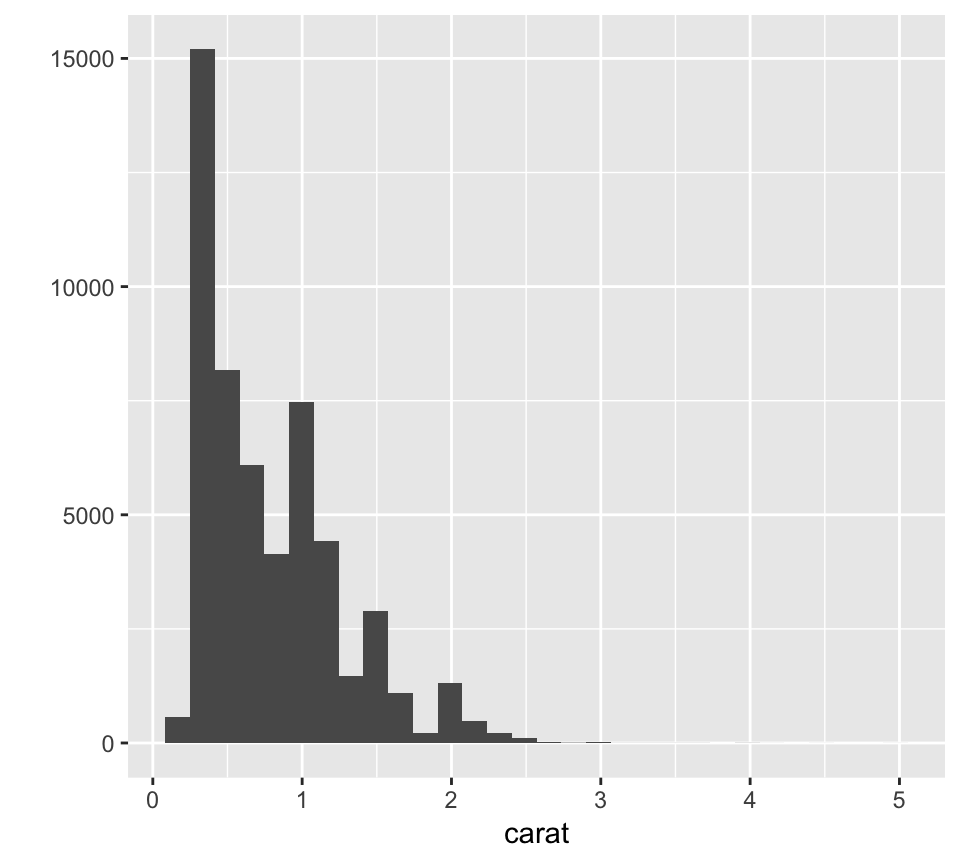## Bivariate Plot

If we specify an additional variable for the y axis, then the default `geom` is a scatter of points.

This time we will also specify a value for `main` which is the main title.

`qplot(x=carat, y=price, data=diamonds, main="Diamond Weight by Price")`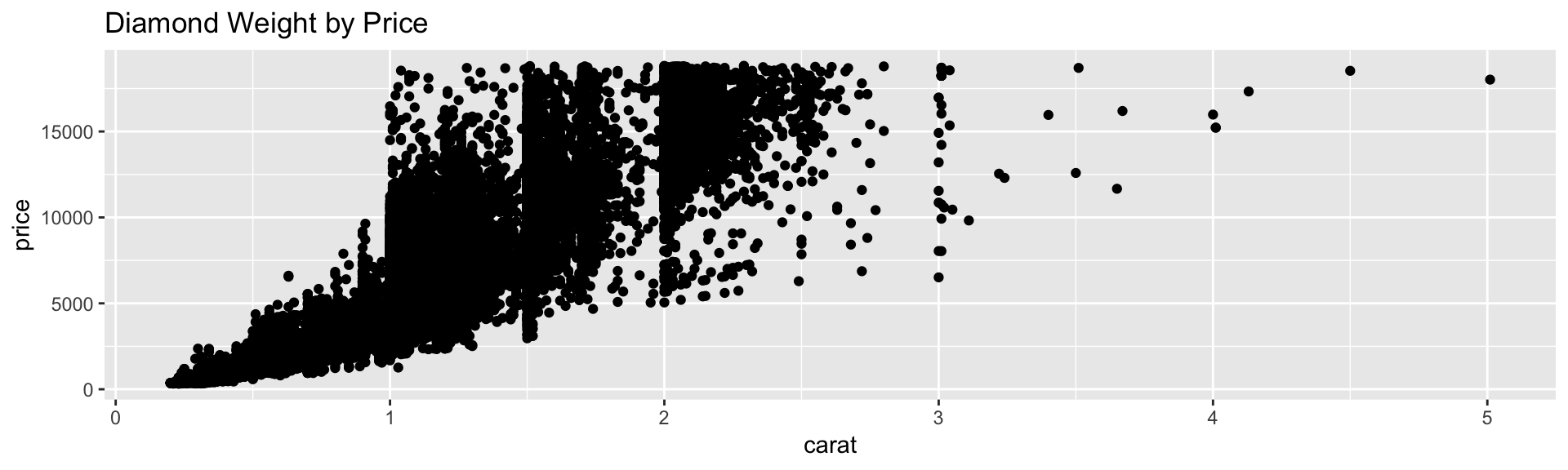## Boxplot

Now lets plot clarity by carat.

`qplot(x=clarity, y=carat, data=diamonds)`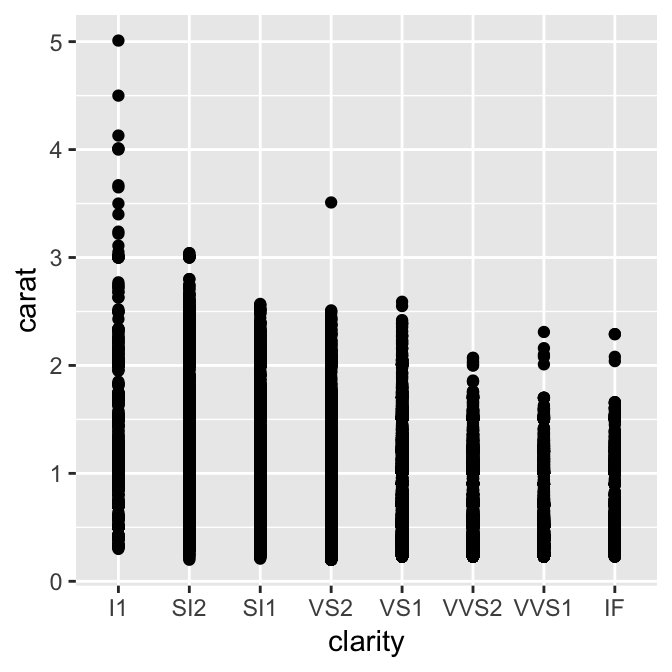Need to specify our `geom`.

We ask for `geom='boxplot'`

```qplot(x=clarity, y=carat,
data=diamonds, geom="boxplot")```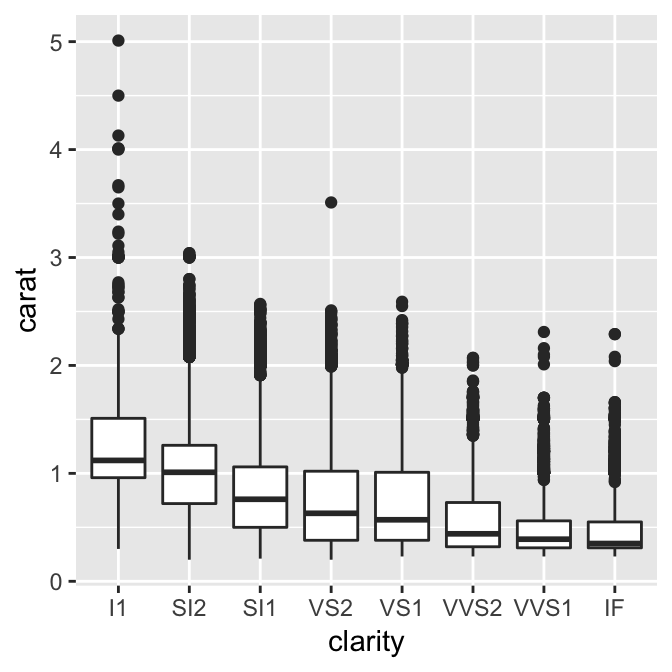## Layers

```weight_by_price <- qplot(x=carat, y=price, data=diamonds, main="Diamond Weight by Price")

weight_by_price + stat_smooth(method="lm")```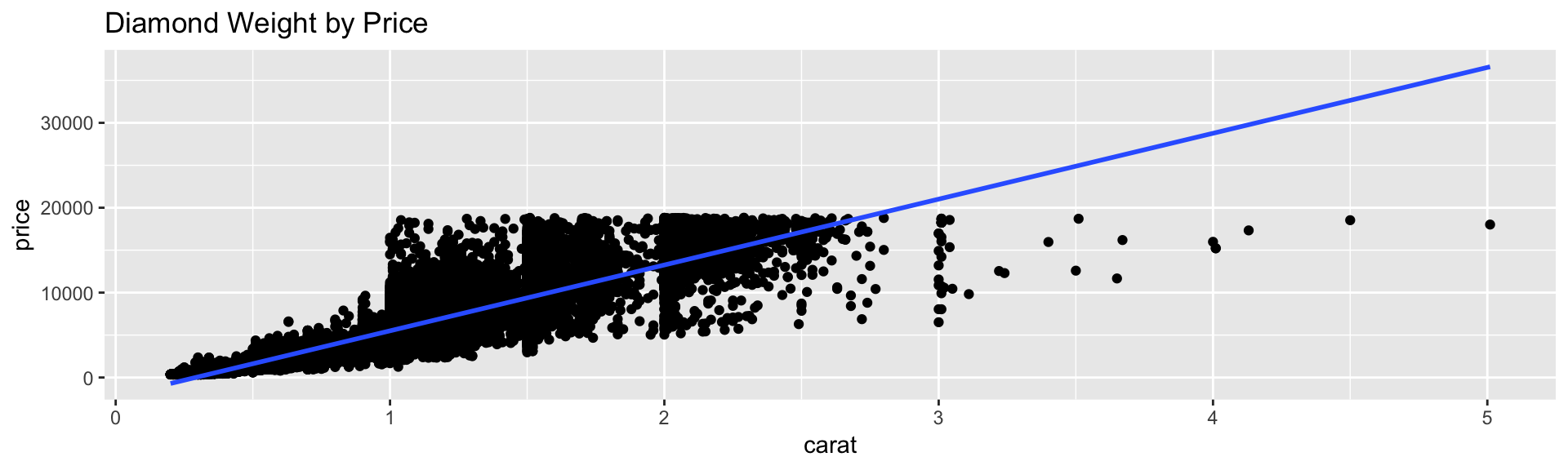## Challenge

The relationship on the previous plot is clearly not linear.

Make the same plot, but with both variables logged.

Don't save logged variables to a variable, log the variables on-the-fly when you make the plot.

## Color, Size, Shape, Fill

Visualize a third variable by changing:

• color or fill
• shape or size of points
```qplot(x=carat, y=price,
data=diamonds, color=clarity)```